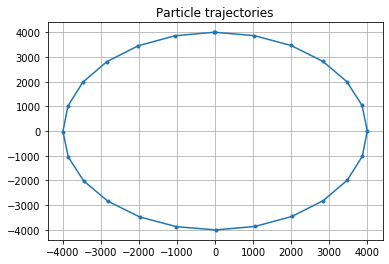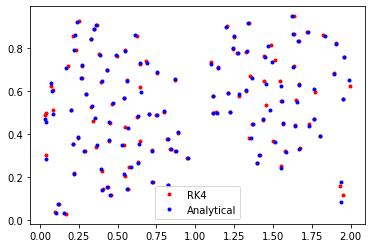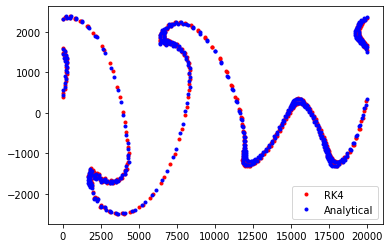## Tutorial on the Analytical Advection kernel in Parcels¶

While Lagrangian Ocean Analysis has been around since at least the 1980s, the Blanke and Raynaud (1997) paper has really spurred the use of Lagrangian particles for large-scale simulations. In their 1997 paper, Blanke and Raynaud introduce the so-called Analytical Advection scheme for pathway integration. This scheme has been the base for the Ariane and TRACMASS tools. We have also implemented it in Parcels, particularly to facilitate comparison with for example the Runge-Kutta integration scheme.

In this tutorial, we will briefly explain what the scheme is and how it can be used in Parcels. For more information, see for example Döös et al (2017).

Most advection schemes, including for example Runge-Kutta schemes, calculate particle trajectories by integrating the velocity field through time-stepping. The Analytical Advection scheme, however, does not use time-stepping. Instead, the trajectory within a grid cell is analytically computed assuming that the velocities change linearly between grid cells. This yields Ordinary Differential Equations for the time is takes to cross a grid cell in each direction. By solving these equations, we can compute the trajectory of a particle within a grid cell, from one face to another. See Figure 2 of Van Sebille et al (2018) for a schematic comparing the Analytical Advection scheme to the fourth order Runge-Kutta scheme.

Note that the Analytical scheme works with a few limitations:

1. The velocity field should be defined on a C-grid (see also the Parcels NEMO tutorial).

And specifically for the implementation in Parcels

1. The AdvectionAnalytical kernel only works for Scipy Particles.
2. Since Analytical Advection does not use timestepping, the dt parameter in pset.execute() should be set to np.inf. For backward-in-time simulations, it should be set to -np.inf.
3. For time-varying fields, only the 'intermediate timesteps' scheme (section 2.3 of Döös et al 2017) is implemented. While there is also a way to also analytically solve the time-evolving fields (section 2.4 of Döös et al 2017), this is not yet implemented in Parcels.

We welcome contributions to the further development of this algorithm and in particular the analytical time-varying case. See here for the code of the AdvectionAnalytical kernel.

Below, we will show how this AdvectionAnalytical kernel performs on one idealised time-constant flow and two idealised time-varying flows: a radial rotation, the time-varying double-gyre as implemented in e.g. Froyland and Padberg (2009) and the Bickley Jet as implemented in e.g. Hadjighasem et al (2017).

First import the relevant modules.

In :
%pylab inline
from parcels import FieldSet, ParticleSet, ScipyParticle, JITParticle, Variable
import numpy as np
from datetime import timedelta as delta
import matplotlib.pyplot as plt

Populating the interactive namespace from numpy and matplotlib


As in Figure 4a of Lange and Van Sebille (2017), we define a circular flow with period 24 hours, on a C-grid

In :
def radialrotation_fieldset(xdim=201, ydim=201):
# Coordinates of the test fieldset (on C-grid in m)
a = b = 20000  # domain size
lon = np.linspace(-a/2, a/2, xdim, dtype=np.float32)
lat = np.linspace(-b/2, b/2, ydim, dtype=np.float32)
dx, dy = lon-lon, lat-lat

# Define arrays R (radius), U (zonal velocity) and V (meridional velocity)
U = np.zeros((lat.size, lon.size), dtype=np.float32)
V = np.zeros((lat.size, lon.size), dtype=np.float32)
R = np.zeros((lat.size, lon.size), dtype=np.float32)

def calc_r_phi(ln, lt):
return np.sqrt(ln**2 + lt**2), np.arctan2(ln, lt)

omega = 2 * np.pi / delta(days=1).total_seconds()
for i in range(lon.size):
for j in range(lat.size):
r, phi = calc_r_phi(lon[i], lat[j])
R[j, i] = r
r, phi = calc_r_phi(lon[i]-dx/2, lat[j])
V[j, i] = -omega * r * np.sin(phi)
r, phi = calc_r_phi(lon[i], lat[j]-dy/2)
U[j, i] = omega * r * np.cos(phi)

data = {'U': U, 'V': V, 'R': R}
dimensions = {'lon': lon, 'lat': lat}
fieldset = FieldSet.from_data(data, dimensions, mesh='flat')
fieldset.U.interp_method = 'cgrid_velocity'
fieldset.V.interp_method = 'cgrid_velocity'
return fieldset



Now simulate a set of particles on this fieldset, using the AdvectionAnalytical kernel. Keep track of how the radius of the Particle trajectory changes during the run.

In :
def UpdateR(particle, fieldset, time):
particle.radius = fieldset.R[time, particle.depth, particle.lat, particle.lon]

class MyParticle(ScipyParticle):

pset = ParticleSet(fieldsetRR, pclass=MyParticle, lon=0, lat=4e3, time=0)

runtime=delta(hours=24),
dt=np.inf,  # needs to be set to np.inf for Analytical Advection
output_file=output)

WARNING: Particle initialisation from field can be very slow as it is computed in scipy mode.


Now plot the trajectory and calculate how much the radius has changed during the run.

In :
output.close()Particle radius at start of run 4000.000000
Particle radius at end of run 4002.483887


### Double-gyre example¶

Define a double gyre fieldset that varies in time

In :
def doublegyre_fieldset(times, xdim=51, ydim=51):
"""Implemented following Froyland and Padberg (2009), 10.1016/j.physd.2009.03.002"""
A = 0.25
delta = 0.25
omega = 2 * np.pi

a, b = 2, 1  # domain size
lon = np.linspace(0, a, xdim, dtype=np.float32)
lat = np.linspace(0, b, ydim, dtype=np.float32)
dx, dy = lon-lon, lat-lat

U = np.zeros((times.size, lat.size, lon.size), dtype=np.float32)
V = np.zeros((times.size, lat.size, lon.size), dtype=np.float32)

for i in range(lon.size):
for j in range(lat.size):
x1 = lon[i]-dx/2
x2 = lat[j]-dy/2
for t in range(len(times)):
time = times[t]
f = delta * np.sin(omega * time) * x1**2 + (1-2 * delta * np.sin(omega * time)) * x1
U[t, j, i] = -np.pi * A * np.sin(np.pi * f) * np.cos(np.pi * x2)
V[t, j, i] = np.pi * A * np.cos(np.pi * f) * np.sin(np.pi * x2) * (2 * delta * np.sin(omega * time) * x1 + 1 - 2 * delta * np.sin(omega * time))

data = {'U': U, 'V': V}
dimensions = {'lon': lon, 'lat': lat, 'time': times}
allow_time_extrapolation = True if len(times) == 1 else False
fieldset = FieldSet.from_data(data, dimensions, mesh='flat', allow_time_extrapolation=allow_time_extrapolation)
fieldset.U.interp_method = 'cgrid_velocity'
fieldset.V.interp_method = 'cgrid_velocity'
return fieldset

fieldsetDG = doublegyre_fieldset(times=np.arange(0, 3.1, 0.1))


Now simulate a set of particles on this fieldset, using the AdvectionAnalytical kernel

In :
X, Y = np.meshgrid(np.arange(0.15, 1.85, 0.1), np.arange(0.15, 0.85, 0.1))
psetAA = ParticleSet(fieldsetDG, pclass=ScipyParticle, lon=X, lat=Y)

output = psetAA.ParticleFile(name='doublegyreAA.nc', outputdt=0.1)
dt=np.inf,  # needs to be set to np.inf for Analytical Advection
runtime=3,
output_file=output)


And then show the particle trajectories in an animation

In :
output.close()
plotTrajectoriesFile('doublegyreAA.nc', mode='movie2d_notebook')

Out:

Now, we can also compute these trajectories with the AdvectionRK4 kernel

In :
psetRK4 = ParticleSet(fieldsetDG, pclass=JITParticle, lon=X, lat=Y)

INFO: Compiled JITParticleAdvectionRK4 ==> /var/folders/r2/8593q8z93kd7t4j9kbb_f7p00000gr/T/parcels-504/cbcb799aee13754c9eae2649e4002775_0.so


And we can then compare the final locations of the particles from the AdvectionRK4 and AdvectionAnalytical simulations

In :
plt.plot(psetRK4.lon, psetRK4.lat, 'r.', label='RK4')
plt.plot(psetAA.lon, psetAA.lat, 'b.', label='Analytical')
plt.legend()
plt.show()The final locations are similar, but not exactly the same. Because everything else is the same, the difference has to be due to the different kernels. Which one is more correct, however, can't be determined from this analysis alone.

### Bickley Jet example¶

Let's as a second example, do a similar analysis for a Bickley Jet, as detailed in e.g. Hadjighasem et al (2017).

In :
def bickleyjet_fieldset(times, xdim=51, ydim=51):
"""Bickley Jet Field as implemented in Hadjighasem et al 2017, 10.1063/1.4982720"""
U0 = 0.06266
L = 1770.
r0 = 6371.
k1 = 2 * 1 / r0
k2 = 2 * 2 / r0
k3 = 2 * 3 / r0
eps1 = 0.075
eps2 = 0.4
eps3 = 0.3
c3 = 0.461 * U0
c2 = 0.205 * U0
c1 = c3 + ((np.sqrt(5)-1)/2.) * (k2/k1) * (c2 - c3)

a, b = np.pi*r0, 7000.  # domain size
lon = np.linspace(0, a, xdim, dtype=np.float32)
lat = np.linspace(-b/2, b/2, ydim, dtype=np.float32)
dx, dy = lon-lon, lat-lat

U = np.zeros((times.size, lat.size, lon.size), dtype=np.float32)
V = np.zeros((times.size, lat.size, lon.size), dtype=np.float32)
P = np.zeros((times.size, lat.size, lon.size), dtype=np.float32)

for i in range(lon.size):
for j in range(lat.size):
x1 = lon[i]-dx/2
x2 = lat[j]-dy/2
for t in range(len(times)):
time = times[t]

f1 = eps1 * np.exp(-1j * k1 * c1 * time)
f2 = eps2 * np.exp(-1j * k2 * c2 * time)
f3 = eps3 * np.exp(-1j * k3 * c3 * time)
F1 = f1 * np.exp(1j * k1 * x1)
F2 = f2 * np.exp(1j * k2 * x1)
F3 = f3 * np.exp(1j * k3 * x1)
G = np.real(np.sum([F1, F2, F3]))
G_x = np.real(np.sum([1j * k1 * F1, 1j * k2 * F2, 1j * k3 * F3]))
U[t, j, i] = U0 / (np.cosh(x2/L)**2) + 2 * U0 * np.sinh(x2/L) / (np.cosh(x2/L)**3) * G
V[t, j, i] = U0 * L * (1./np.cosh(x2/L))**2 * G_x

data = {'U': U, 'V': V, 'P': P}
dimensions = {'lon': lon, 'lat': lat, 'time': times}
allow_time_extrapolation = True if len(times) == 1 else False
fieldset = FieldSet.from_data(data, dimensions, mesh='flat', allow_time_extrapolation=allow_time_extrapolation)
fieldset.U.interp_method = 'cgrid_velocity'
fieldset.V.interp_method = 'cgrid_velocity'
return fieldset

fieldsetBJ = bickleyjet_fieldset(times=np.arange(0, 1.1, 0.1)*86400)


Add a zonal halo for periodic boundary conditions in the zonal direction

In :
fieldsetBJ.add_constant('halo_west', fieldsetBJ.U.grid.lon)

def ZonalBC(particle, fieldset, time):
if particle.lon < fieldset.halo_west:
particle.lon += fieldset.halo_east - fieldset.halo_west
elif particle.lon > fieldset.halo_east:
particle.lon -= fieldset.halo_east - fieldset.halo_west


And simulate a set of particles on this fieldset, using the AdvectionAnalytical kernel

In :
X, Y = np.meshgrid(np.arange(0, 19900, 100), np.arange(-100, 100, 100))

psetAA = ParticleSet(fieldsetBJ, pclass=ScipyParticle, lon=X, lat=Y, time=0)

output = psetAA.ParticleFile(name='bickleyjetAA.nc', outputdt=delta(hours=1))
dt=np.inf,
runtime=delta(days=1),
output_file=output)


And then show the particle trajectories in an animation

In :
output.close()
plotTrajectoriesFile('bickleyjetAA.nc', mode='movie2d_notebook')

Out:

Like with the double gyre above, we can also compute these trajectories with the AdvectionRK4 kernel

In :
psetRK4 = ParticleSet(fieldsetBJ, pclass=JITParticle, lon=X, lat=Y)
dt=delta(minutes=5), runtime=delta(days=1))

INFO: Compiled JITParticleAdvectionRK4ZonalBC ==> /var/folders/r2/8593q8z93kd7t4j9kbb_f7p00000gr/T/parcels-504/2c3dc13dcb5affb33a37376ddd7e7666_0.so


And finally, we can again compare the end locations from the AdvectionRK4 and AdvectionAnalytical simulations

In :
plt.plot(psetRK4.lon, psetRK4.lat, 'r.', label='RK4')
plt.plot(psetAA.lon, psetAA.lat, 'b.', label='Analytical')
plt.legend()
plt.show()